# 莫凡Python学习笔记——PyTorch 动态神经网络（五）：卷积神经网络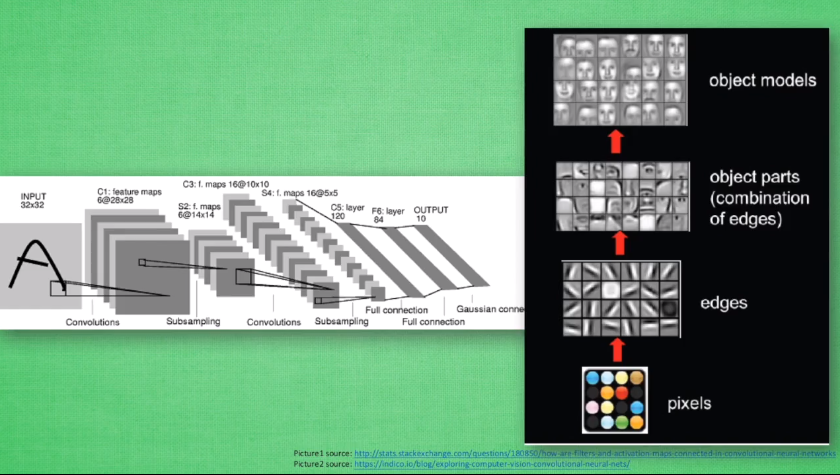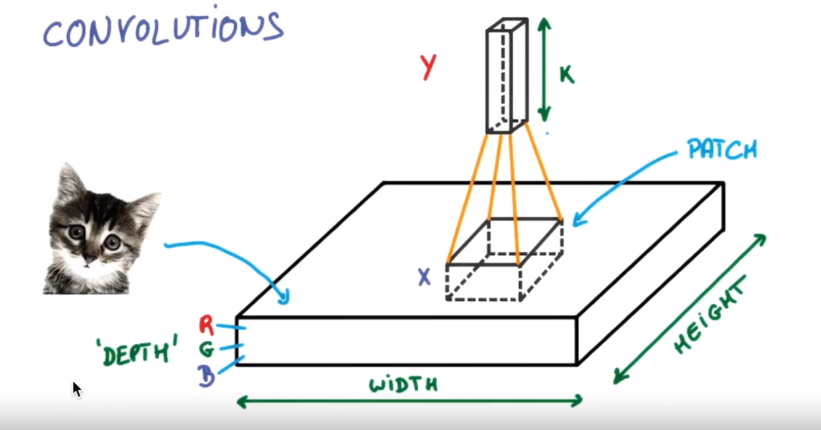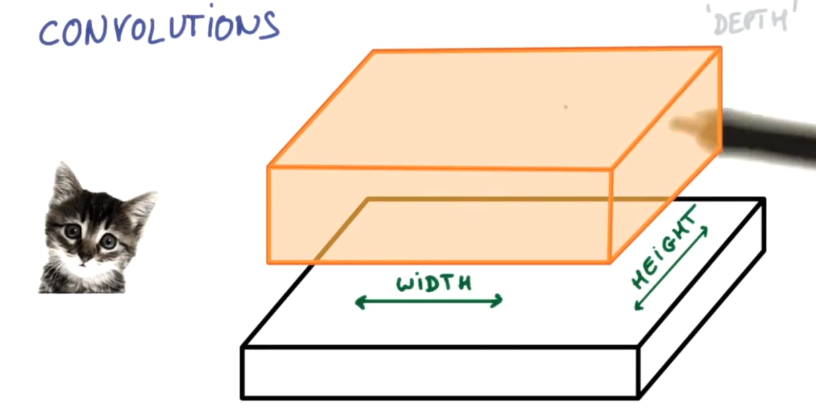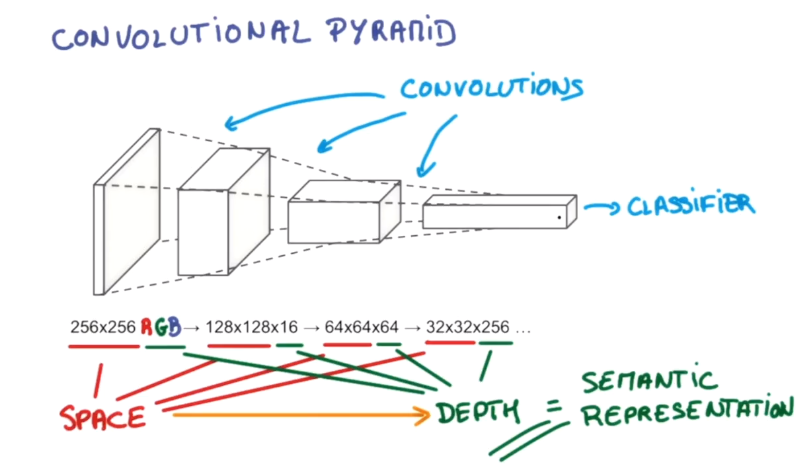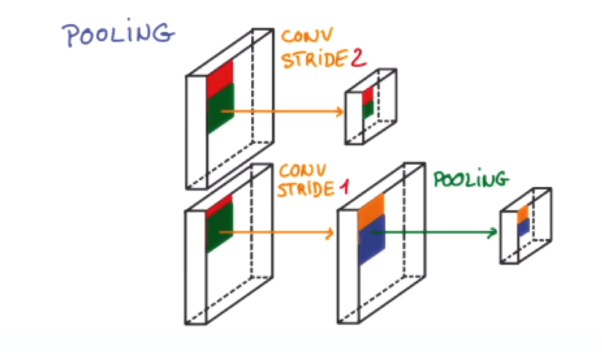import torch
import torch.nn as nn
import torch.utils.data as Data
import torchvision
import matplotlib.pyplot as plt

#Hyper Parameters
EPOCH = 1     #train the traning data n times, to save time, we just train 1 epoch
BATCH_SIZE = 50
LR = 0.001    #learning rate

train_data = torchvision.datasets.MNIST(
root = './minst',
train = True,    #if the train is True,the data downloaded is train data, however，it is test data. and test data is little than train data.
transform=torchvision.transforms.ToTensor(),#改变数据类型(0,1)，适合CNN输入
)
print (train_data.train_data.size())
print (train_data.train_labels.size())
plt.imshow(train_data.train_data.numpy(),cmap='gray')
plt.title('%i' % train_data.train_labels)
plt.show()

test_data = torchvision.datasets.MNIST(root='./mnist/',train=False)   #train=False  表示提取出来的是test_data
test_x = Variable(torch.unsqueeze(test_data.test_data, dim=1),volatile=True).type(torch.FloatTensor)[:2000]/255.   #提取测试数据并转换维度和格式,除以255，手动压缩到（0,1）
test_y = test_data.test_labels[:2000]  #都只是选取了钱2000个

#构建CNN网络
class CNN(nn.Module):
def __init__(self):
super(CNN,self).__init__()
self.conv1 = nn.Sequential(
nn.Conv2d(          #(1,28,28)
in_channels=1,   #输入的数据的高度，这里用的是黑白图像所以是1，如果是彩色图像，那高度应该是3
out_channels=16,    #输出的高度，也就是filter（过滤器）的个数，几个filter同时在一个区域进行扫描提取，然后将提取的信息放入下一层进行分析
kernel_size=5,  #filter是5*5的大小
stride=1,    #隔多少跳一下，步长，也就是每步跳多大间隔

),    #卷积层，只是一个有长宽高的过滤器，长宽表示获取信息的范围，高大概表示获取信息的量的意思吧。(16,28,28)
nn.ReLU(),      #神经网络   (16,28,28)
nn.MaxPool2d(kernel_size=2), #池化层,也就是用大小为2的pooling的手段，相当于把图片进行裁剪，成长宽更小但是高度不变的图片   (16,14,14)
)
self.conv2 = nn.Sequential(
nn.Conv2d(16,32,5,1,2),    #(32,14,14)
nn.ReLU(),              #(32,14,14)
nn.MaxPool2d(2)   #pooling 有两种，一种是最大的，一种是中值得，一般使用最大值的      (32,7,7)
)
self.out = nn.Linear(32 * 7 * 7, 10)   #这里需要二维的数据，但是上面conv2之后是三维的，所以需要将三维的数据展成二维的，在forWord来做

def forward(self, x):
x = self.conv1(x)
x = self.conv2(x)            #(batch,32,7,7)考虑了batch
x = x.view(x.size(0),-1)      #扩展展平   (batch,32*7*7)
output = self.out(x)
return output, x
cnn = CNN()
print (cnn)
#定义优化器和损失函数
loss_func = nn.CrossEntropyLoss()
#训练
for epoch in range(EPOCH):
b_x = Variable(x)
b_y = Variable(y)

output = cnn(b_x)
loss = loss_func(output, b_y)
loss.backward()
optimizer.step()

if step % 50 == 0:
test_output = cnn(test_x)    #进行测试
pred_y = torch.max(test_output, 1).data.squeeze()
accuracy = sum(pred_y == test_y) / test_y.size(0)
print ('Epoch: ', epoch, '| train loss: %.4f' %loss.data, '| test accuracy: ')
test_output = cnn(test_x[:10])  #取前10个数据
pred_y = torch.max(test_output, 1).data.numpy().squeeze()
print(pred_y, 'prediction number')
print(test_y[:10].numpy(), 'real number')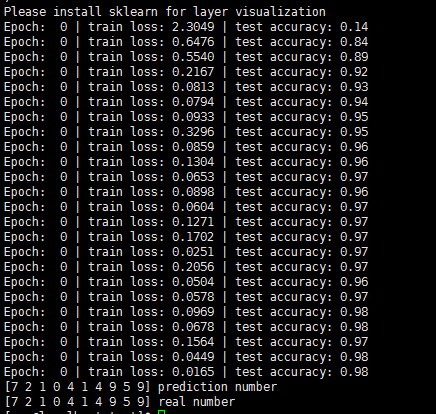©️2019 CSDN 皮肤主题: 编程工作室 设计师: CSDN官方博客